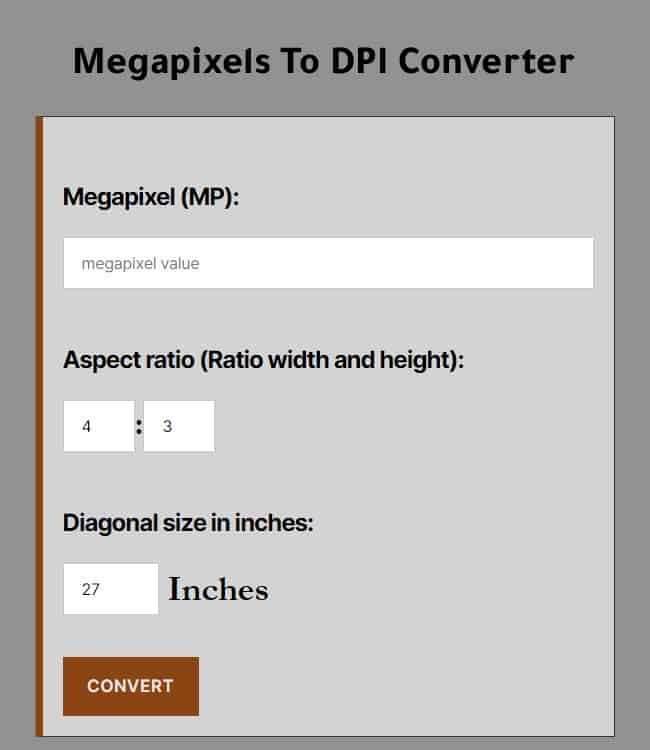# Megapixels To DPI Converter

#### Megapixels To DPI Converter

This is a free and easy-to-use converter that you can use to convert megapixels to dpi (dots per inch) accurately.

:

##### Diagonal size in inches:

Inches

Megapixel is one million (1,000,000) pixels that form an image. megapixels are one of the important characteristics of a monitor and a digital camera. Also in megapixels, the size of the created or scanned image is measured to correlate its size with the size of a known image.

The aspect ratio of an image is the proportional ratio of width to height. You know it as two numbers separated by a colon in x:y format.

#### How to use megapixels to dpi converter

First,  enter the megapixel value

Second,  enter the aspect ratio value

Third,  enter the diagonal value in inches

Finally,  click on the convert button

#### Manually and online megapixels to dpi conversion

You can convert megapixels (MP) to dpi either manually or online.

To convert MP to dpi manually you need the following formulas:

First formula to calculate width in pixels:

Width in pixel = √(Aspect ratio * megapixel value * 1000000)

Second formula to calculate height in pixels:

Height in pixel = √(1/Aspect ratio * megapixel value * 1000000)

Third formula to calculate diagonal in pixels:

Diagonal In Pixels = √(width² + height²)

Finally, calculate the dpi value:

DPI = Diagonal In Pixels/Diagonal In Inches

To convert megapixels to dpi online, you can use the above converter for fast and accurate conversion.#### Megapixels to dpi conversion chart

This is a table for most common megapixels to dpi conversion results at 4:3 aspect ratio and 27 inches diagonal.

Megapixels DPI
1 Megapixels 53.46 DPI
2.07 Megapixels 76.91 DPI
6 Megapixels 130.95 DPI
6.1 Megapixels 132.03 DPI
7.1 Megapixels 142.44 DPI
8 Megapixels 151.2 DPI
10 Megapixels 169.05 DPI
10.1 Megapixels 169.89 DPI
12.8 Megapixels 191.26 DPI